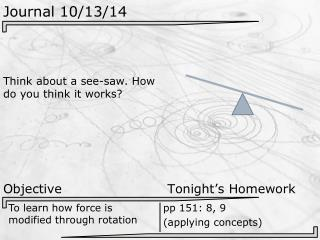DownloadDownload PresentationJournal 10/13/14

# Journal 10/13/14

Télécharger la présentation## Journal 10/13/14

- - - - - - - - - - - - - - - - - - - - - - - - - - - E N D - - - - - - - - - - - - - - - - - - - - - - - - - - -
##### Presentation Transcript

1. Journal 10/13/14 Think about a see-saw. How do you think it works? Objective Tonight’s Homework To learn how force is modified through rotation pp 151: 8, 9 (applying concepts)

2. Finishing Projectile Motion Let’s wrap up projectile motion by going over any last practice problems people want explained.

3. Demo on Torque Mr. C. is going to do a demo up front on something called “torque”. While he does this demo, pay close attention. There’s a physical law being modeled here that you may be able to figure out. Afterwards, we’re going to take some notes.

4. Notes on Torque We’ve seen that if we apply a force to an object, that object can move. But our demo has shown us that if we have something rotating, the amount of movement varies depending on where we apply force. More movement Less movement

5. Notes on Torque Somehow, distance has something to do with getting objects to rotate. Angle seems to matter as well. Most Some Little movement movementmovement

6. Equations Torque E: V: S: τ = r F sin(θ) τ = Torque (measured in N●m) r = the distance along the lever arm from the pivot to the point of force (in m) F = The amount of force being applied (in N) sin(θ) = the angle between the lever arm and the force applied This equation tells us the ability of a force to move something around a pivot point. While not strictly a force, this more or less equates to a “rotational force”.

7. Notes on Torque Example: A bolt attached to a piece of metal has become stuck. If it requires a torque of 600 Nm to turn freely, what length of lever arm must be used if the person turning can exert a perpendicular force of 300 N?

8. Notes on Torque Example: A bolt attached to a piece of metal has become stuck. If it requires a torque of 600 Nm to turn freely, what length of lever arm must be used if the person turning can exert a perpendicular force of 300 N? τ = r F sin(θ) 600 Nm = (r)(300 N) (sin 90) r = 600 Nm / [(300 N) (sin 90)] r = 600 Nm / 300 N r = 2 m

9. Notes on Torque Example: A string is attached to a 1.6 m long wrench. If the string is pulled at an angle of 27°, whattorque will result from a forceof 250 N? 27° 1.6 m

10. Notes on Torque Example: A string is attached to a 1.6 m long wrench. If the string is pulled at an angle of 27°, whattorque will result from a forceof 250 N? τ = r F sin(θ) τ = (1.6 m)(250 N)(sin 27) τ = (400 N m)(0.454) τ = 181.6 N m 27° 1.6 m

11. Exit Question #22 What would happen if you applied a direct force to a bolt instead of a torque? a) The bolt could pull from what it’s stuck in b) The bolt could snap off or break c) The bolt could turn, but slower d) The bolt could turn, but faster e) The bolt could explode f) None of the above